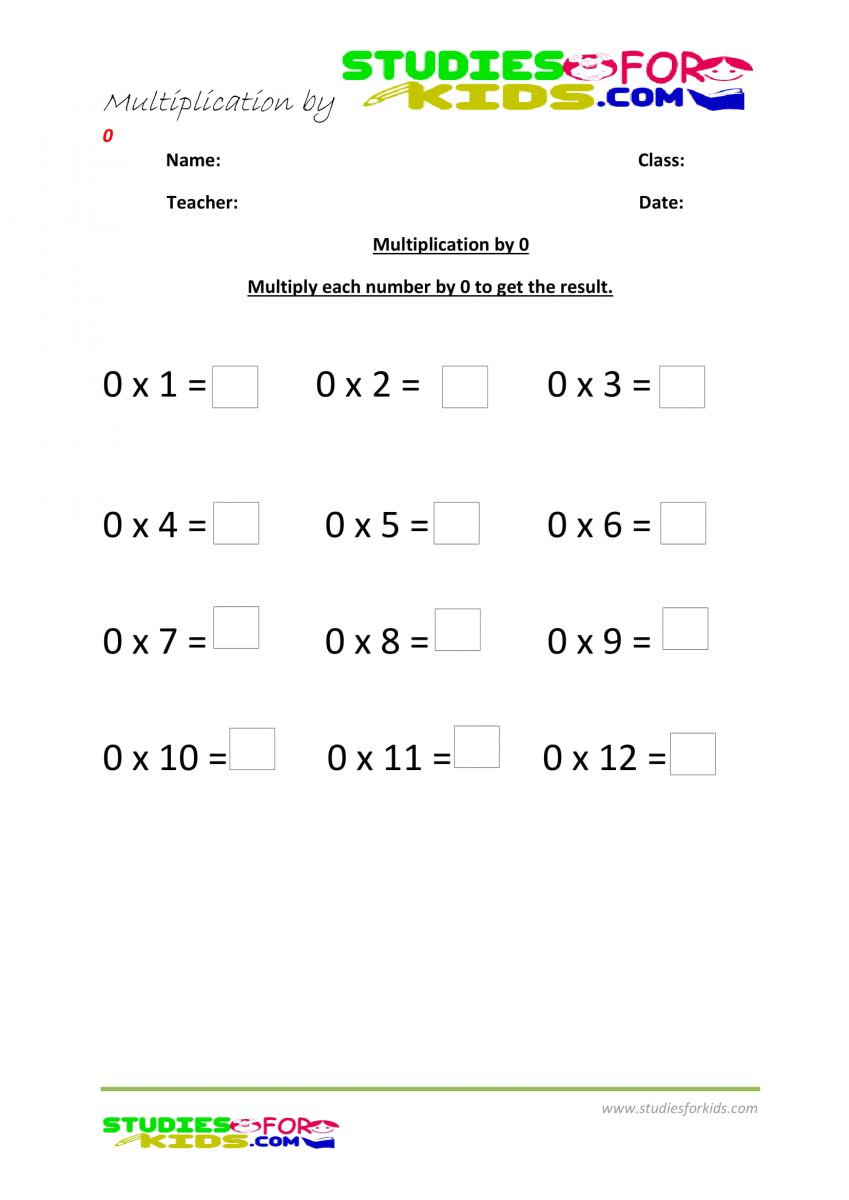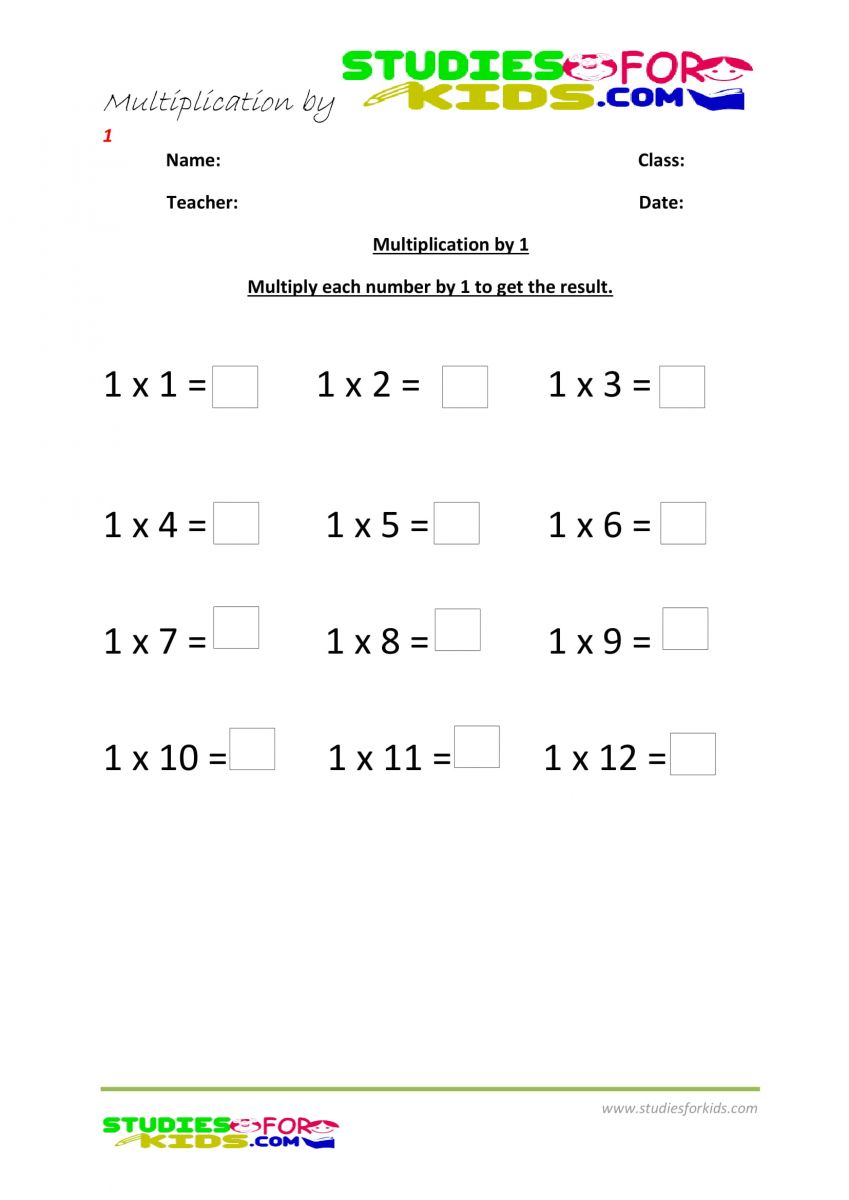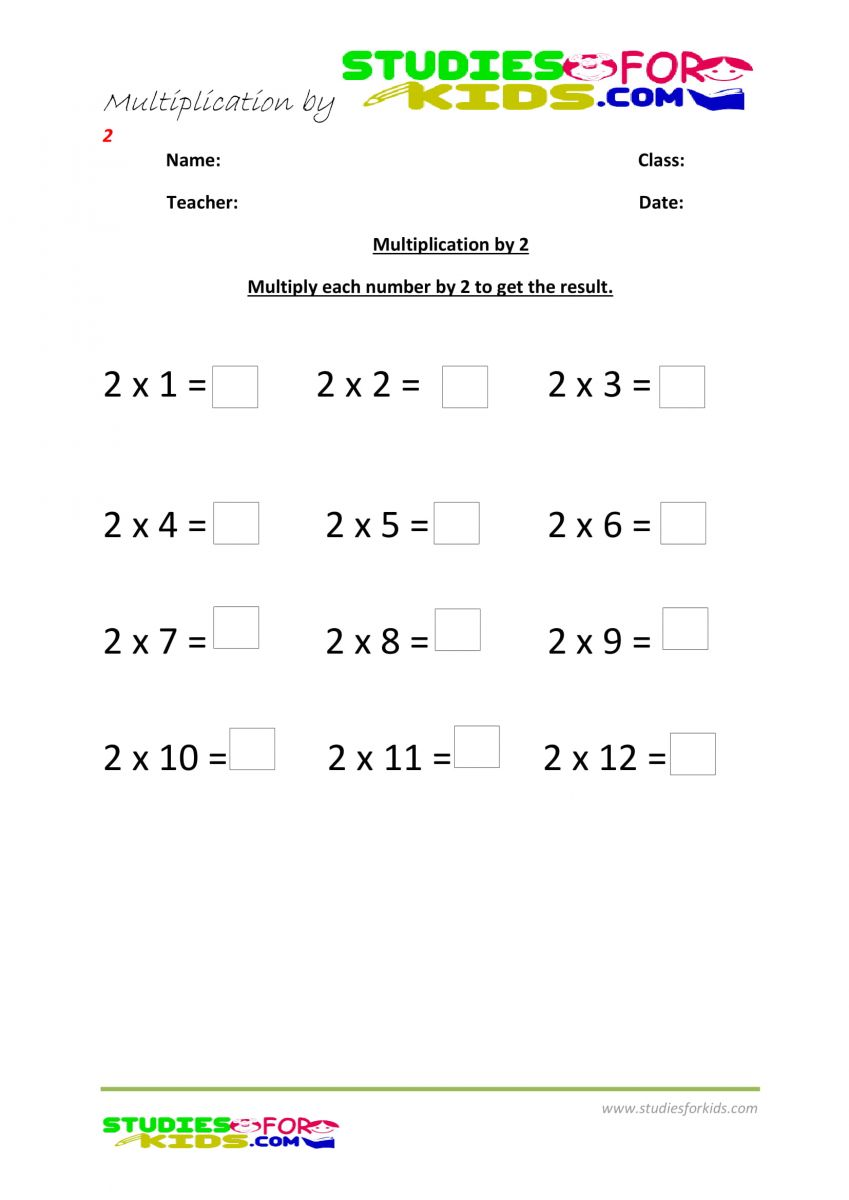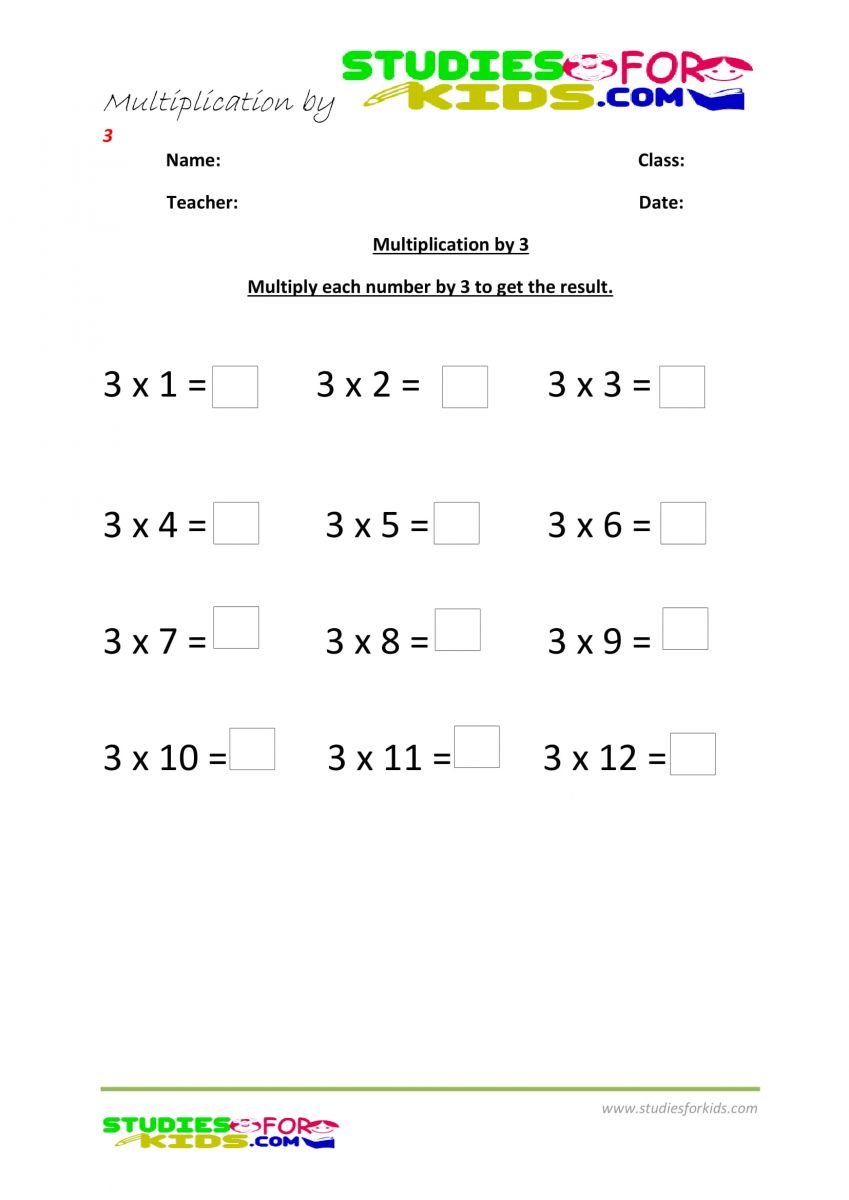# Math Worksheets for Grade 2

In this page you will find math worksheets for grade 2 on math topics such as addition, multiplication,substration ,

• Multi-Digit Numbers
• Decimal Numbers
• Decimal Numbers and the Tenths Place
• Decimal Numbers and the Hundredths Place
• Decimal Numbers and the Thousandths Place
• Rounding Decimal Numbers
• Comparing Decimal
• Exponents and Powers of Ten
• Converting Numerical Expressions to Different Forms
• Understanding Expressions and Equations
• Solving Basic Algebraic Equations
• Analyze Arithmetic Patterns